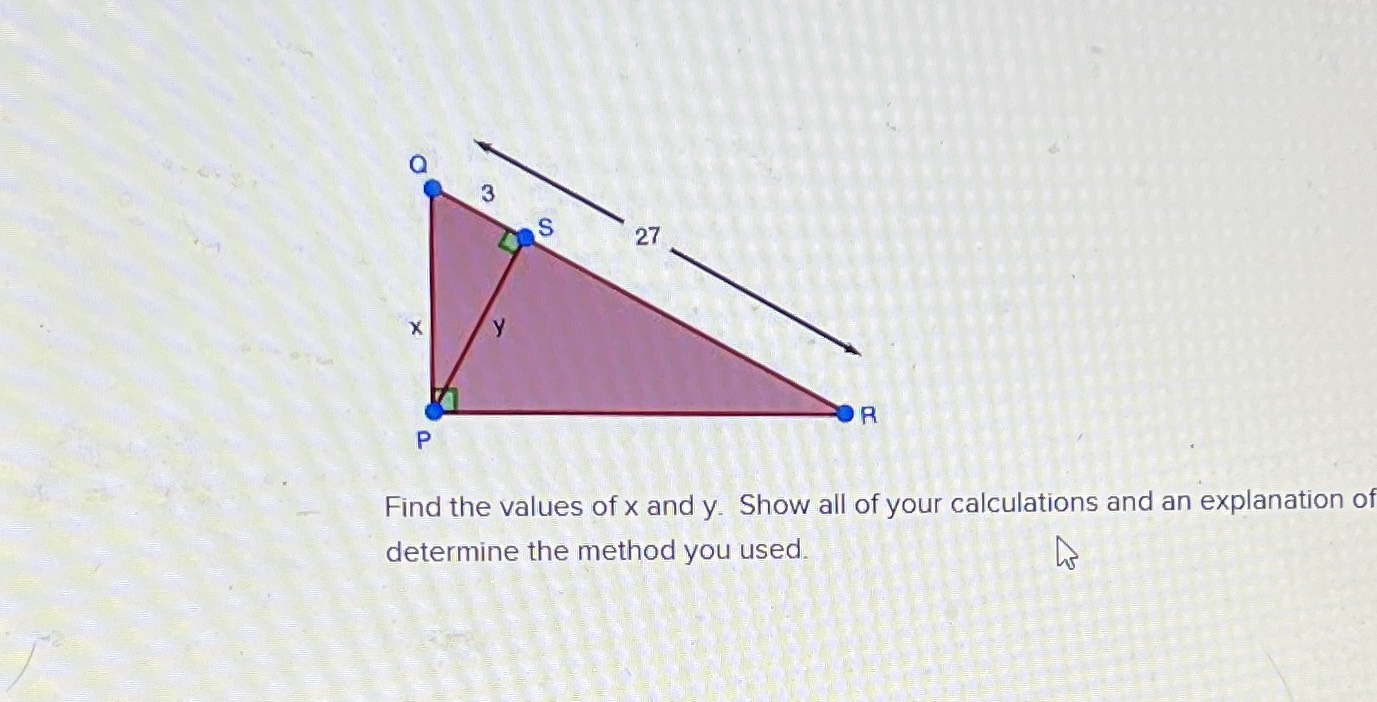### ¿Todavía tienes preguntas de matemáticas?

Pregunte a nuestros tutores expertos
Trigonometry
PreguntaFind the values of $$x$$ and $$y$$ . Show all of your calculations and an explanation of determine the method you used.

y=6$$\sqrt{2}$$